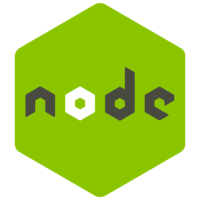This lesson is for members. Join us? Get access to all 3,000+ tutorials + a community with expert developers around the world.

Unlock This Lesson
1×
Become a member
to unlock all featuresLevel Up!

Access all courses & lessons on egghead today and lock-in your price for life.

Autoplay

Classify JSON text data with machine learning in NaturalNatural
^0.4.0

In this lesson, we will learn how to train a Naive Bayes classifier and a Logistic Regression classifier - basic machine learning algorithms - on JSON text data, and classify it into categories.

While this dataset is still considered a small dataset -- only a couple hundred points of data -- we'll start to get better results.

The general rule is that Logistic Regression will work better than Naive Bayes, but only if there is enough data. Since this is still a pretty small dataset, Naive Bayes works better here. Generally, Logistic Regression takes longer to train as well.

This uses data from Ana Cachopo: http://ana.cachopo.org/datasets-for-single-label-text-categorizationCodeYou must be a Member to view code

Access all courses and lessons, track your progress, gain confidence and expertise.

Become a Member
and unlock code for this lessonDiscussTranscript

As always, we'll import our natural library. We'll also import the fs module, because we'll be working with the file system. We'll also make a new classifier by saying new natural.BayesClassifier.

Here, we're going to try to make a classifier that categorizes Internet comments as either related to science and space or politics. Behind the scenes, I have two JSON files that have several hundred examples in both the training and test data.

First, we're going to import our training data. We'll do that by saying fs.readFile, our training data file name and encoding and a callback. If there's an error, we'll log it out. Otherwise, we'll say var trainingData = JSON.parse(data). We'll pass that data to a train function that we'll make down here.

This will take our training data. Because training sometimes takes a while, it's helpful to make indicators to ourselves to know that it's working. Here, we'll just say that we started this training function.

Then, we'll add all of training data to the classifier by doing trainingData.forEach passing the item and saying classifier.addDocument that item text and the item label.

Once all the training data's been added, we can train the classifier by saying classifier.train. We're also going to add time indicators here so that we can see how long it takes to train. We'll log that out.

We'll call the loadTestData function here. Here, we'll make a new function loadTestData. We'll make another indicator to ourselves. We'll do the same thing we did in loading the file above fs.readFile('test_data.json), an encoding and our callback.

If there is an error, then, we'll log that out. Otherwise, we'll say testData = JSON.parse(data). We'll pass that into a function called testClassifier. To do that, we'll say function testClassifier. We'll make an indicator to ourselves.

In order to see how good the classifier is, we need to get a sense of what percentage of labels it accurately categorizes on data it's never seen before. To do that, we'll create a variable here numCorrect and set that to 0That way, when we're running through our test data, we can make a variable called labelGuess which is the label that the classifier guesses for this item's text. Then, compare if the classifier's guess is equal to the item's actual label, then number correct increases by 1.

After all the test data has been classified, we can say the correct percentage is equal to the number correct over the number of test data points. Let's see how this fits together.

We'll fast forward a little bit here, but we can see that training took about 25 seconds. Here, we can see that the classifier predicted categories with about 88.6 percent accuracy.

Natural also provides support for a logistic regression classifier. The code is exactly the same except you replace Bayse classifier with LogisticRegression classifier.

Here, we can see that LogisticRegression classifier predicted categories with about 85.65 percent accuracy. In this case, the naive Bayse classifier performed better.